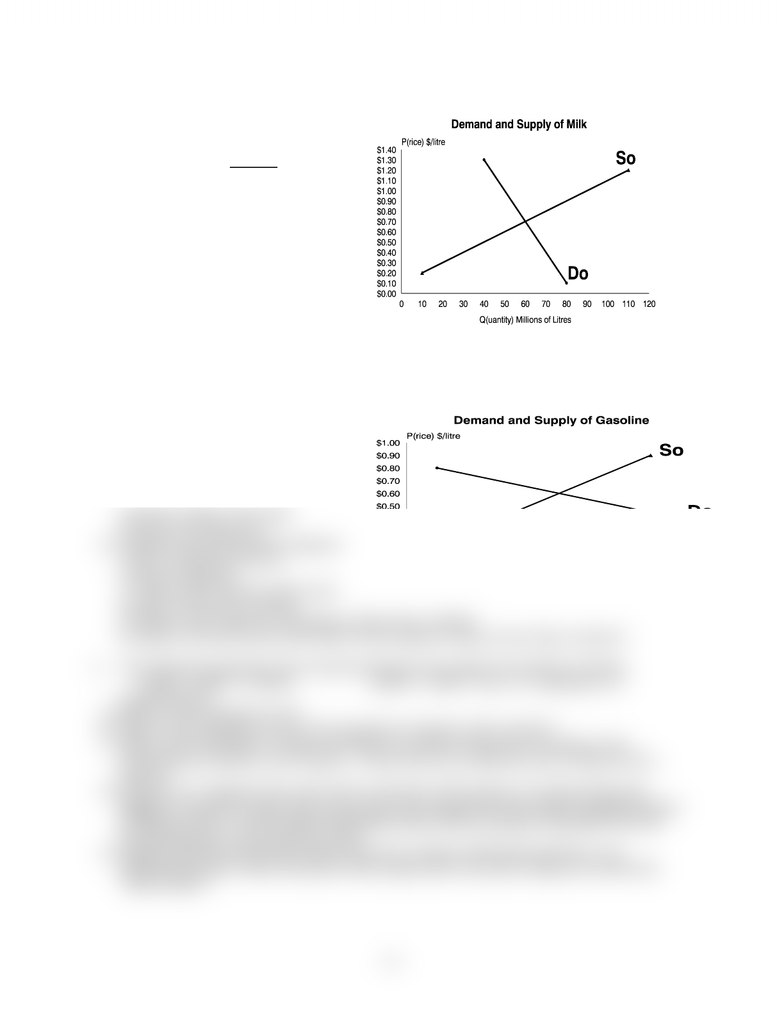Textbook Notes (270,000)
CA (160,000)
WLU (8,000)
BU (2,000)
BU472 (9)
Chapter 4

BU472 Chapter Notes - Chapter 4: Price Ceiling, Price Floor, Economic Equilibrium

Department
Course Code
BU472
Professor
Theresa Romkey
Chapter
4

This preview shows half of the first page. to view the full 2 pages of the document.Problem Set #3: Market Equilibrium and Government Intervention
1. A demand and supply situation for
milk is given in the accompanying
diagram. Prices are in \$/Litre
quantities are in millions of litres.
a/ What is the equilibrium price and
quantity of milk?
b/ What is the total expenditure by
consumers at this equilibrium?
c/ Suppose that government imposes a price
floor of \$1.00/litre for milk to sustain
small farm production by promising to
i/ What is the quantity demanded and
the quantity supplied of milk at this
price?
ii/ What is the amount of milk sold in the market? What is the amount of the surplus
or shortage of milk at this price?
iii/ What must the government do to maintain this price floor in the face of market
forces? What is the cost to the government of this action?
2. The accompanying diagram shows
the demand and supply curves
and initial equilibrium for gasoline.
Prices are in \$/litre and quantities
are in millions of litres.
a) What is the equilibrium price and
quantity? What is the total
revenue of producers?
b) Suppose the government imposes
a price ceiling of \$0.40 to
conserve gasoline.
i) What is the amount sold in the
market at this price ceiling?
ii) What is the surplus or shortage at this price ceiling?
iii) What is the price that will ensue if this output is sold on the 'black market'?
3. The following equaitons give market demand and supply for bushels of wheat.
1000P = 8000 – 0.001Q 1000P = 2000 + 2Q (P = \$/bushel, Q =
bushel/wheat)
a) Which is the demand curve?
b) What is the equilibrium price and quantity of wheat in this market?
c) What is the shortage or surplus of wheat in Canada if the price of wheat in the
international market is \$4 a bushel. (Treat this as an effective price ceiling of \$4 a
bushel.)
d) Indicate on a diagram the cost to the consumer of this policy as measured by the
change in consumer surplus that ensues due to the change from the market equilibrium price
to the price floor; use the quantity demanded rather than the quantity demanded since the
international price causes the price floor.
e) Suppose that the government imposes the price ceiling of \$4/bushel and there is no
international trade. What is the price if the output due to the price ceiling was sold in the
"black market".
- 1 -